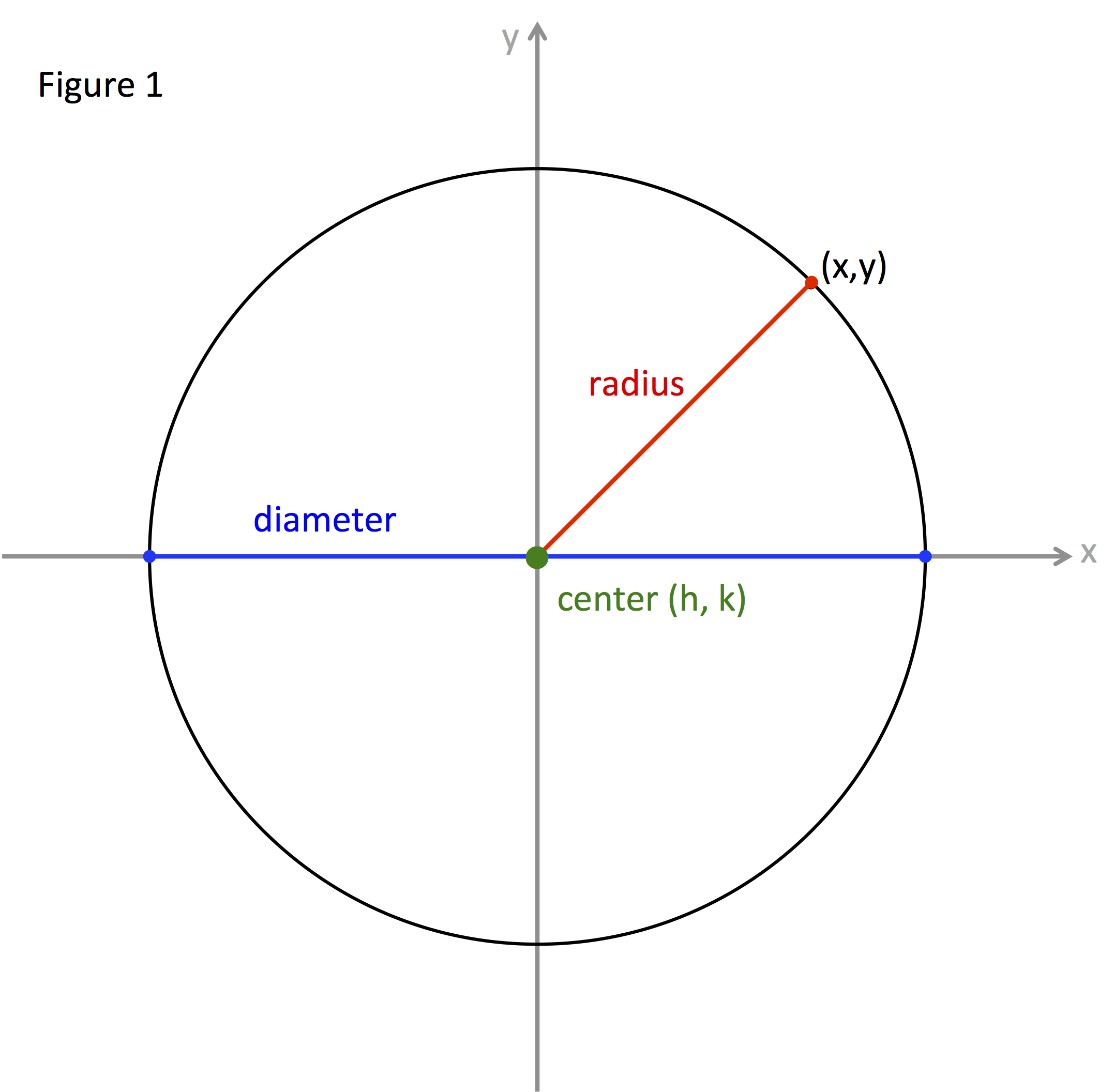A circle is defined as all the points (x, y) in a plane a fixed distance away from a fixed center point.

DEFINITION OF A CIRCLE:

All the points (x, y) in a plane that are equidistant from a fixed center point.

Figure 1 is a circle with the center, radius, and diameter identified.The center is a fixed point in the middle of the circle; usually given the general coordinates (h, k).

The fixed distance from the center to any point on the circle is called the radius.

A line segment from one point on the circle to another point on the circle that passes through the center is twice the radius in length. This line segment is called the diameter of the circle.

A circle can be represented by two different forms of equations, the general form and the center-radius form. This discussion will focus on the center-radius form.

Let the center be (h, k) and the radius be r.

${\left(x-h\right)}^{2}+{\left(y-k\right)}^{2}={r}^{2}$

Let's use the center-radius equation in some examples:

Example 1: What is the center-radius equation of the circle with a diameter of 12 and a center at (-2, 5)
 Step 1: Identify the center coordinates and the radius. The center coordinates are given in the problem. The radius must be determined from the diameter, which is 2 times the radius or $r=\frac{d}{2}$. Center: (-2, 5) Given $Radius=\frac{diameter}{2}$ $r=\frac{12}{2}\to {r}{=}{6}$ Step 2: Substitute the center coordinates and radius into the center-radius form. Be careful to include negative signs when substituting the center coordinates. ${\left(x-h\right)}^{2}+{\left(y-k\right)}^{2}={r}^{2}$   Original ${\left[x-\left(-{2}\right)\right]}^{2}+{\left(y-{5}\right)}^{2}={{6}}^{2}$ Sub. ${\left(x+2\right)}^{2}+{\left(y-5\right)}^{2}=36$ Simplify
Example 2: Circle G has a radius of 8 and center at the origin. What is the positive x-coordinate of the point on the circle with a y-coordinate of -3?
 Step 1: Write the center-radius equation for the circle. Center: (0, 0) Given Radius = 8  Given ${\left(x-h\right)}^{2}+{\left(y-k\right)}^{2}={r}^{2}$ Original ${\left(x-{0}\right)}^{2}+{\left(y-{0}\right)}^{2}={{8}}^{2}$ Sub. ${x}^{2}+{y}^{2}=64$ Simplify Step 2: Determine the x-coordinate associated with a y-coordinate of -3. Substitute -3 in for y and solve for x. ${x}^{2}+{y}^{2}=64$ Original ${x}^{2}+{\left(-3\right)}^{2}=64$ Sub. ${x}^{2}+9=64$   Square ${x}^{2}=55$      Subtract $x=\sqrt{55}\approx 7.4$   Square Root

 Related Links: Math algebra Circle: General Equation Parabola: Standard Equation Pre Calculus